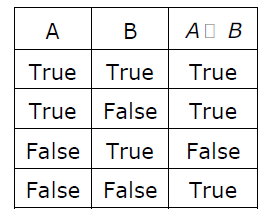Related Articles
GATE | GATE-CS-2006 | Question 28
• Difficulty Level : Basic
• Last Updated : 03 Jul, 2014

A logical binary relation □ ,is defined as follows:Let ~ be the unary negation (NOT) operator, with higher precedence than □.

Which one of the following is equivalent to A∧B ?

```(A) (~A □ B)
(B) ~(A □ ~B)
(C) ~(~A □ ~B)
(D) ~(~A □ B) ```

(A) A
(B) B
(C) C
(D) D

Answer: (D)

Explanation: In A∧B, we have 3 entries as False, and one as True. In table, it is opposite case, so we have to negate A □ B, moreover, we want True only when both A and B are true, so in 3rd entry (which becomes true after negation), we want both true, so we have to negate A also.
So A ∧ B ≡ ~(~A □ B), so option (D) is correct.My Personal Notes arrow_drop_up